### 9.3.3.1 Extending the formulation

Since a game under Formulation 9.7 can be nicely expressed as a matrix, it is tempting to use linear algebra to conveniently express expected costs. Let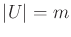and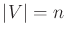. As in Section 9.1.3, a randomized strategy for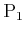can be represented as an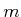-dimensional vector,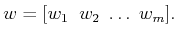(9.55)

The probability axioms of Section 9.1.2 must be satisfied: 1)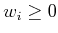for all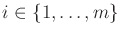, and 2)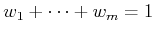. If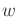is considered as a point in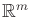, then the two constraints imply that it must lie on an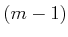-dimensional simplex (recall Section 6.3.1). If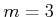, this means thatlies in a triangular subset of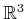. Similarly, let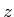represent a randomized strategy for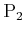as an-dimensional vector,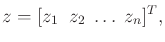(9.56)

that also satisfies the probability axioms. In (9.56),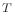denotes transpose, which yields a column vector that satisfies the dimensional constraints required for an upcoming matrix multiplication.

Let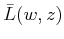denote the expected cost that will be received ifplaysandplays. This can be computed as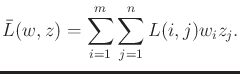(9.57)

Note that the cost,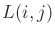, makes use of the assumption in Formulation 9.7 that the actions are consecutive integers. The expected cost can be alternatively expressed using the cost matrix,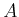. In this case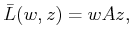(9.58)

in which the product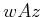yields a scalar value that is precisely (9.57). To see this, first consider the product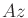. This yields an-dimensional vector in which theth element is the expected cost thatwould receive if it tries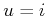. Thus, it appears thatviewsas a nature player under the probabilistic model. Onceandare multiplied, a scalar value is obtained, which averages the costs in the vectoraccording the probabilities of.

Let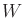and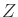denote the set of all randomized strategies forand, respectively. These spaces include strategies that are equivalent to the deterministic strategies considered in Section 9.3.2 by assigning probability one to a single action. Thus,andcan be considered as expansions of the set of possible strategies in comparison to what was available in the deterministic setting. Usingand, randomized security strategies forandare defined as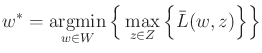(9.59)

and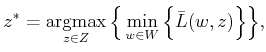(9.60)

respectively. These should be compared to (9.44) and (9.46). The differences are that the space of strategies has been expanded, and expected cost is now used.

The randomized upper value is defined as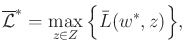(9.61)

and the randomized lower value is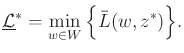(9.62)

Sinceandinclude the deterministic security strategies,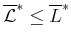and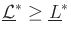. These inequalities imply that the randomized security strategies may have some hope in closing the gap between the two values in general.

The most fundamental result in zero-sum game theory was shown by von Neumann [956,957], and it states that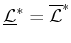for any game in Formulation 9.7. This yields the randomized value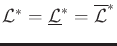for the game. This means that there will never be expected regret if the players stay with their security strategies. If the players apply their randomized security strategies, then a randomized saddle point is obtained. This saddle point cannot be seen as a simple pattern in the matrixbecause it instead exists overand.

The guaranteed existence of a randomized saddle point is an important result because it demonstrates the value of randomization when making decisions against an intelligent opponent. In Example 9.7, it was intuitively argued that randomization seems to help when playing against an intelligent adversary. When playing the game repeatedly with a deterministic strategy, the other player could learn the strategy and win every time. Once a randomized strategy is used, the players will not experience regret.

Steven M LaValle 2020-08-14